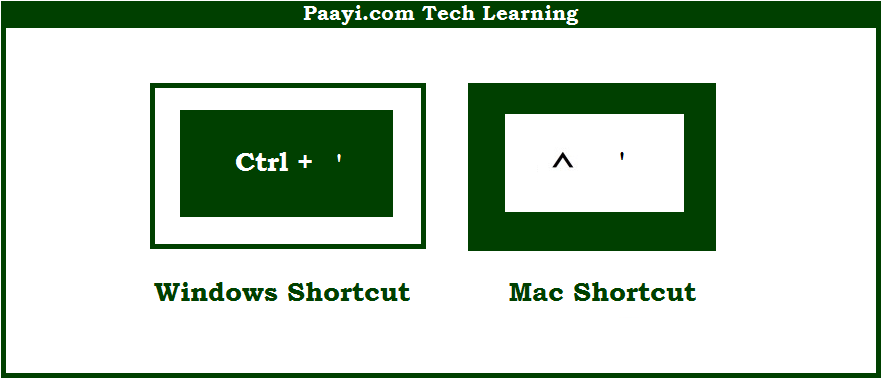# Learn How to Copy Formula From Cell Above With Shortcut in Microsoft Excel

Written by | 0 Comments | 643 Views

In this article, you will learn how to Copy Formula From Cell Above with a shortcut in Microsoft Excel. You will also get to know the shortcut to Copy Formula From Cell Above on Mac. The easy key combinations will make you operate Microsoft Excel smoothly

Copy Formula From Cell Above With Shortcut in Microsoft Excel

Here we will show you how to copy the formula from the cell above in Microsoft Excel. Like many other programs Microsoft Excel also uses similar key combinations while using shortcuts.

You can use the key combinations given below to copy the formula from the cell above using the shortcut. When you use this shortcut, it will copy the formula in the cell above to the active or current cell and leaves the cell in edit mode. This is the easy method to make an exact copy of a formula, cell references are also not changed.

This shortcut leaves the cell in "edit mode" after the copy, so you can immediately edit it. This is helpful when you want to copy a value from above and adjust the result manually. For example, if you need to enter a set of voucher numbers that are similar, but not necessarily sequential. So, here we go:

## How to Copy Formula From Cell Above With Shortcut in Microsoft Excel?It is used to copy the formula from the cell above in Microsoft Excel. When you use this shortcut, it will copy the formula in the cell above to the active or current cell and leaves the cell in edit mode. This is the easy method to make an exact copy of a formula, cell references are also not changed.

This shortcut leaves the cell in "edit mode" after the copy, so you can immediately edit it. This is helpful when you want to copy a value from above and adjust the result manually. For example, if you need to enter a set of voucher numbers that are similar, but not necessarily sequential. This way you can easily copy the formula from the cell above using this shortcut.